### Introduction to Earth Data Analysis

1. Get Started
1. Learn Git/Github for team collaboration
2. Install Python via Anaconda
3. Install Python IDE (Jupyter Notebook, Visual Studio Code)
4. Python variables and data types
5. Install and use Python packages
6. Get familiar with Numpy for data manipulations
7. Get familiar with Matplotlib for plotting arrays
8. Get familiar with Pandas to analyze tabular data
2. Get familiar with text file formats
1. Use basic Markdown syntax to format text in Jupyter Notebook files
2. Get familiar with text file formats - CSV, .txt, YAML
3. Read/Write data from text files using Python
4. Read/Write data from text files using Pandas
5. Deal with missing data in Pandas
3. Spatial Data Analysis
1. Read multi-layered raster data (.tif / .hdf / .nc) in Python
2. Read vector data (shapefiles) using geopandas
3. GIS in Python
4. Time-series Analysis using Pandas
2. Work with `Datetime` formats
3. Resample time series
4. Filtering/smoothing time-series with Pandas
5. Plot time series
5. How to plot topographic high-resolution geospatial maps

## Recent posts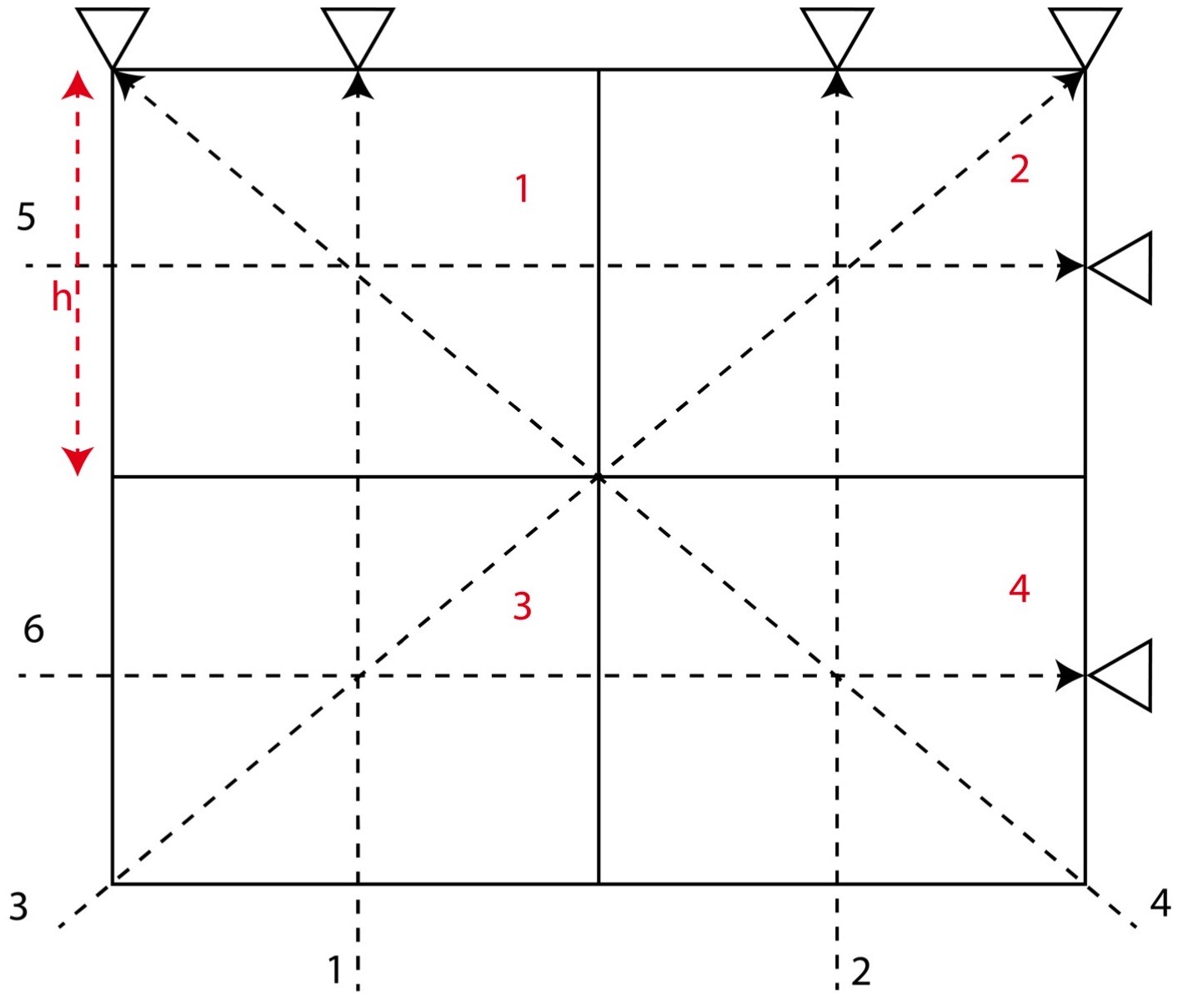## Numerical tests on travel time tomography [matlab]

Introduction to the concepts of tomography with equations and codes. Introduction to the concepts of overdetermined, underdetermined and mix-determined probl...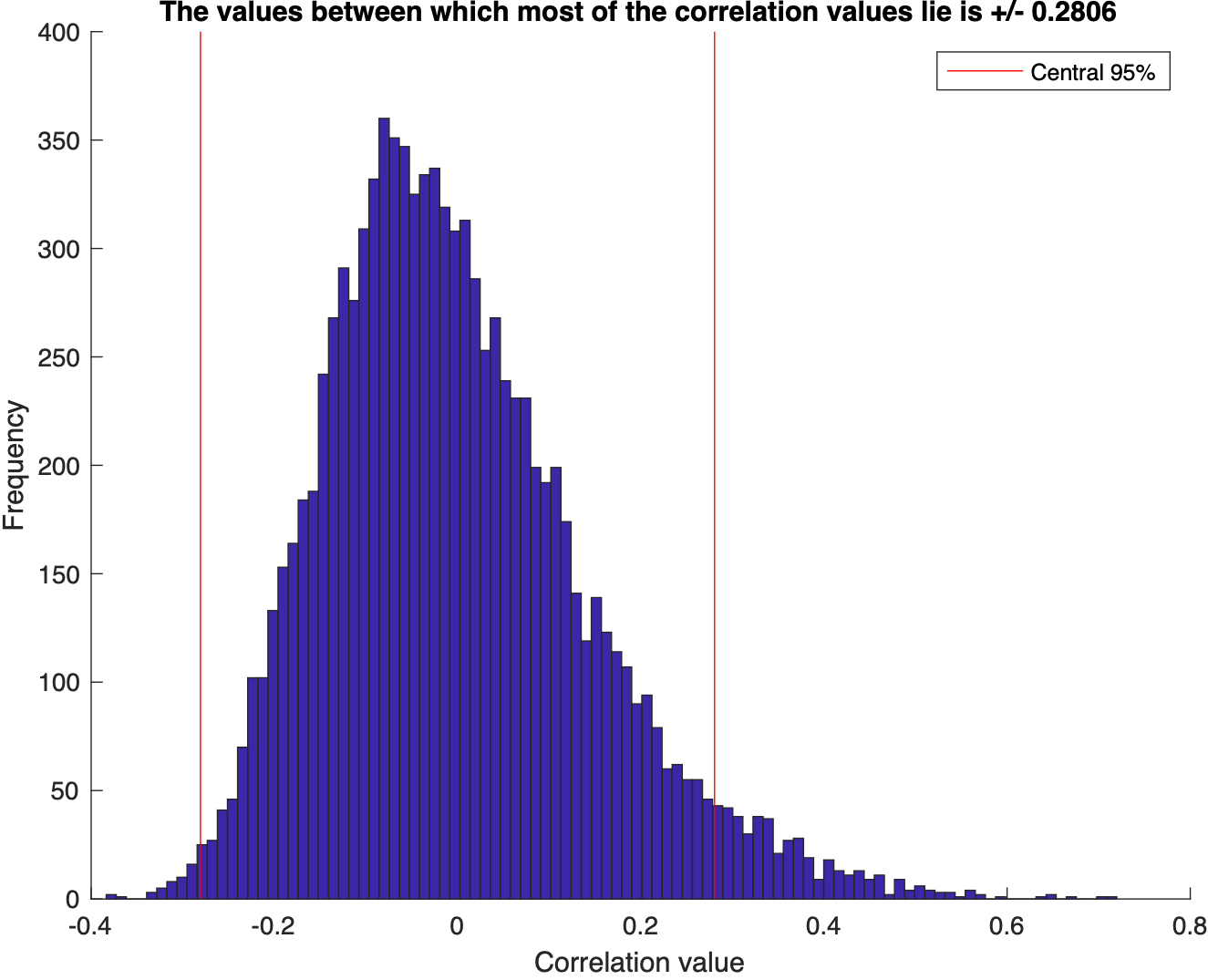## Monte carlo simulation to test for the correlation between two dataset in matlab (codes included)

We test for the correlation coefficients or the covariance between two sets of random numbers selected from normal distribution using the Monte Carlo simulat...## Easy statistical analysis using the tools of matlab (codes included)

Visualize the statistics of the data using MATLAB: mean, median, std, interquartile range, skewness, kurtosis, t-statistic, degrees of freedom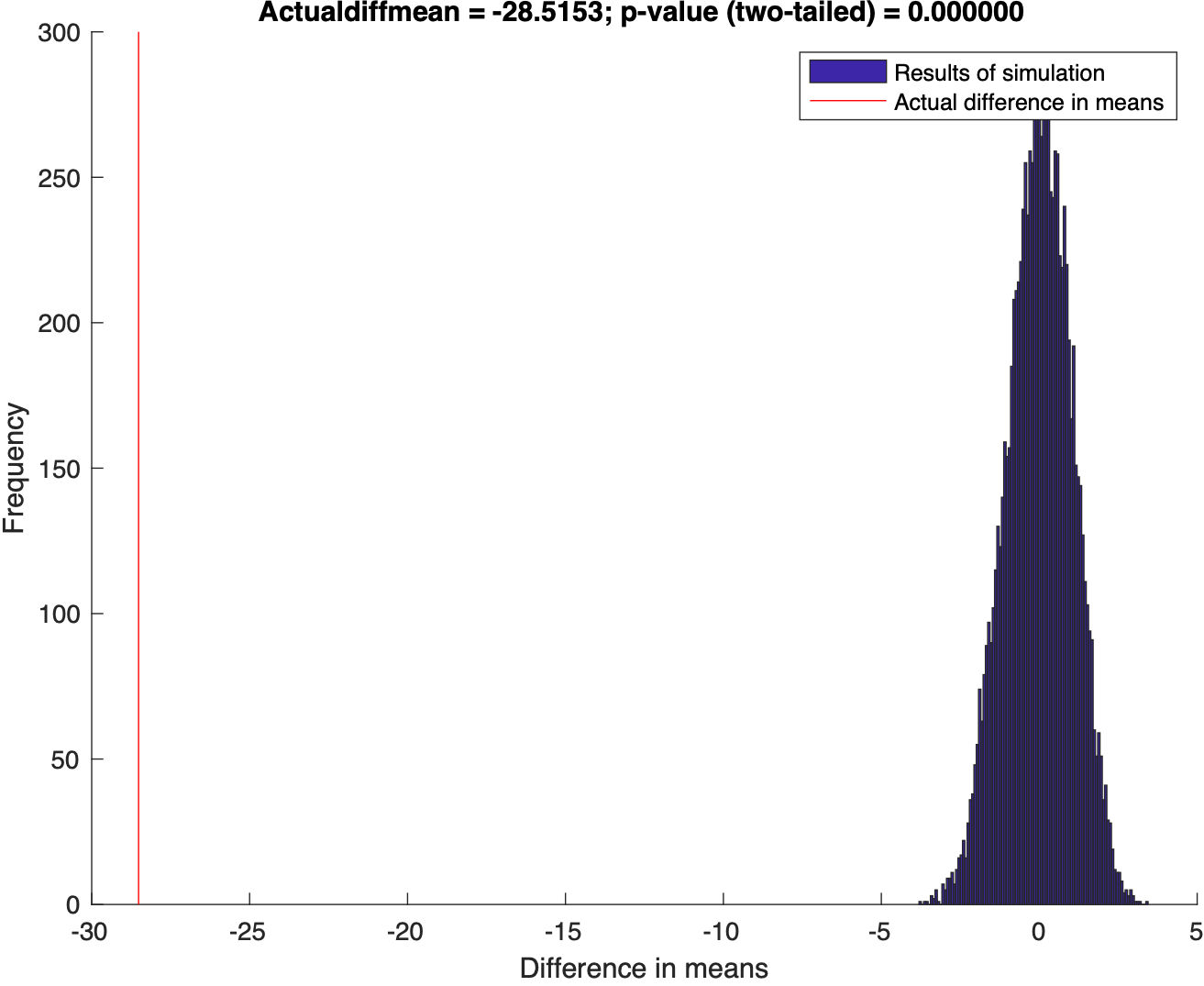## Hypothesis test in matlab (codes included)

Using Randomization to test the disprove the null hypothesis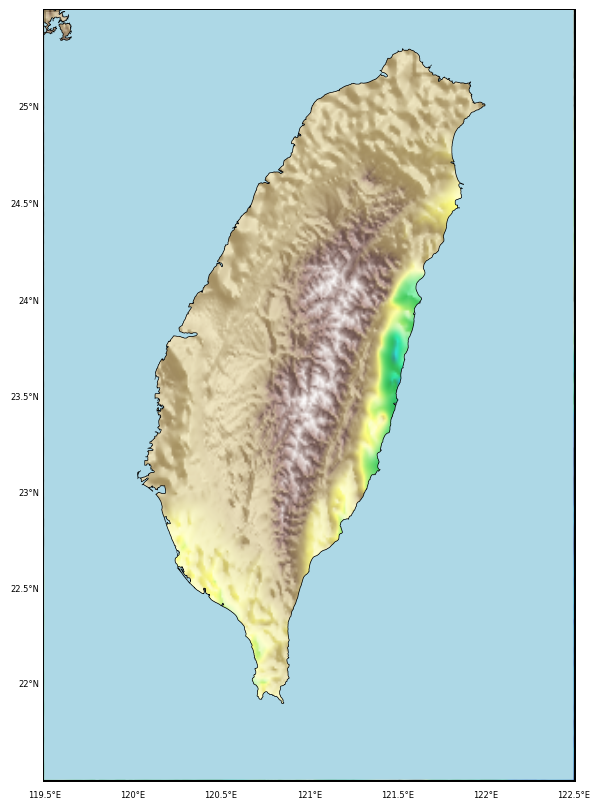## Topographic map clipped by coastlines in python (codes included)

This post demonstrate how to use Python to set up clip topographic map based on coastlines.## Topographic map with shading in python (codes included)

Generating GMT style shaded relief map in Python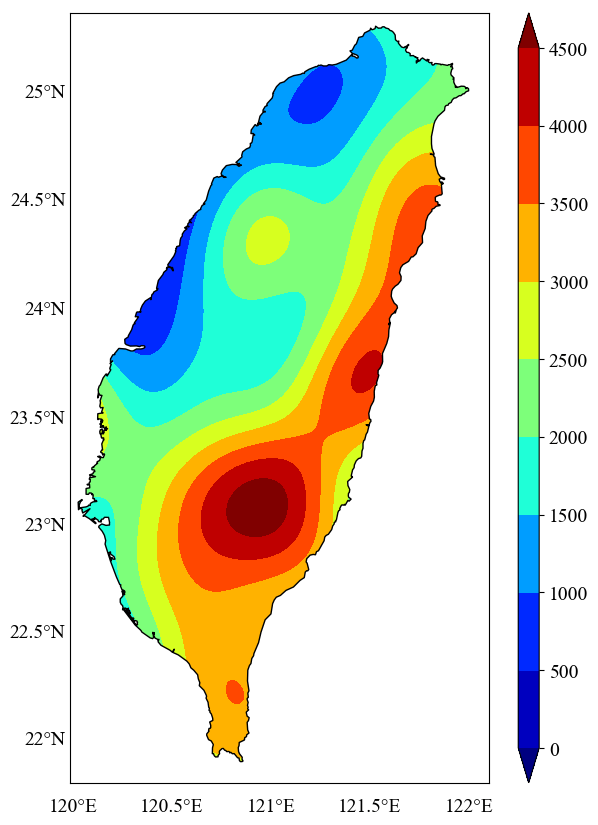## Plotting the geospatial data clipped by coastlines in python (codes included)

In geosciences, we most frequently have to make geospatial plots, but the available data is unevenly distributed and irregular. We like to show the data, in ...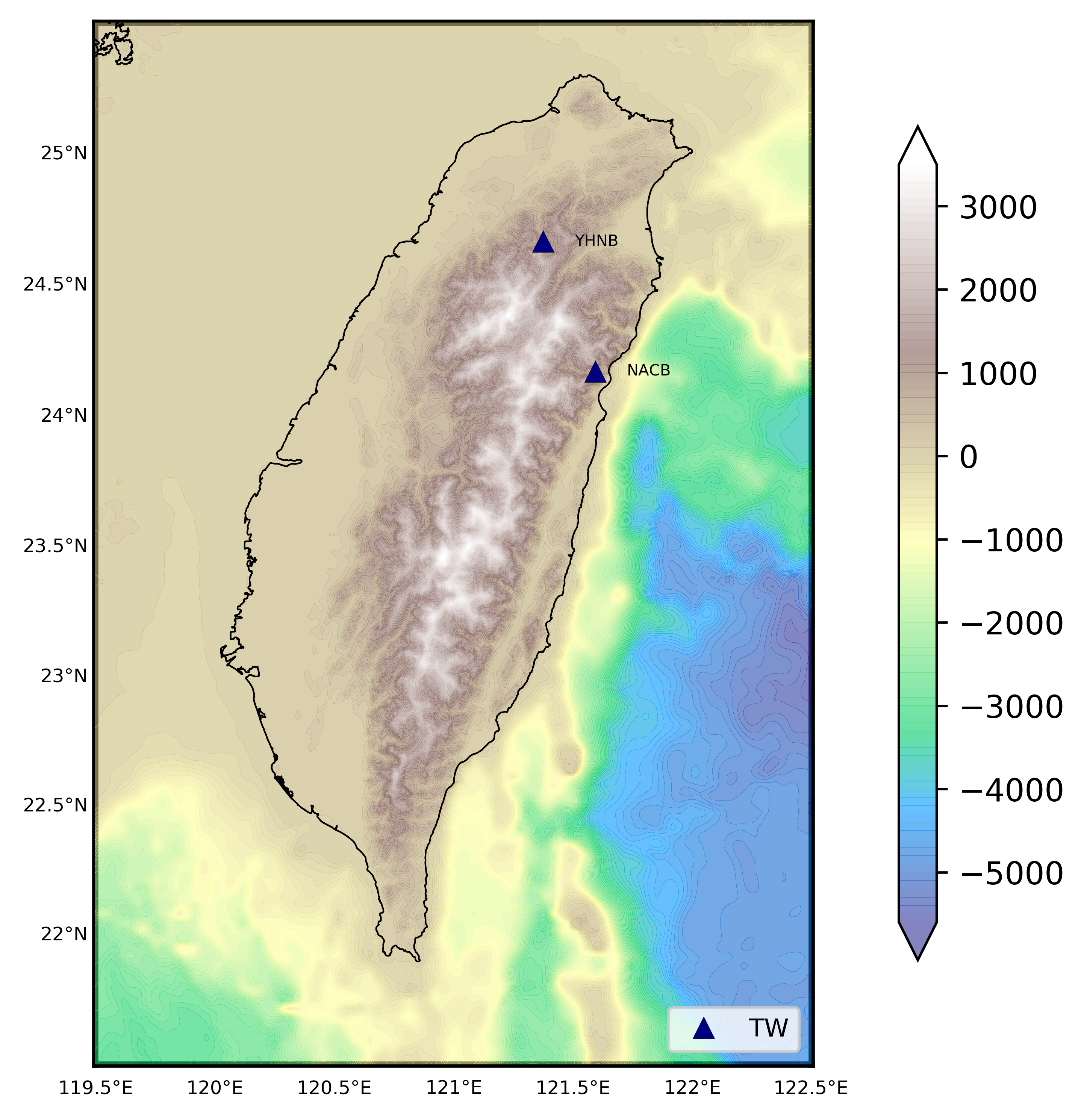## How to plot topographic station map in python (codes included)

We read the station information from a file to plot the 1 arc-minute relief map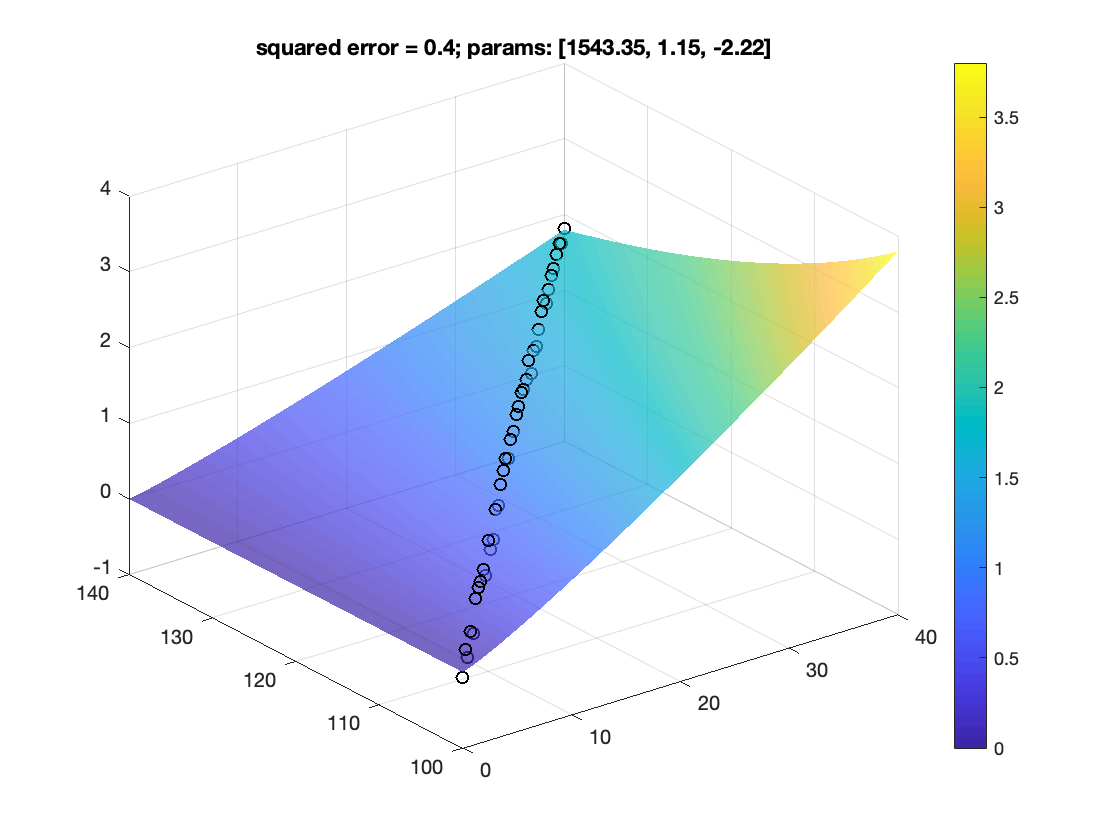## Non-linear curve fitting to a model with multiple observational variables in matlab (codes included)

How to fit data to non-linear model## Plotting 1 arc-minute global relief map in python (codes included)

Plotting 1 arc-minute topographic map in python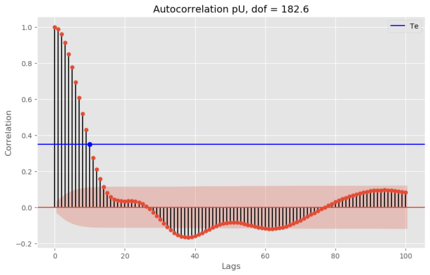## Estimation of the degrees of freedom of time series in python (codes included)

Two time series with predominant linear trends (very low DOF) can have a very high correlation coefficient, which can hardly be construed as an evidence for ...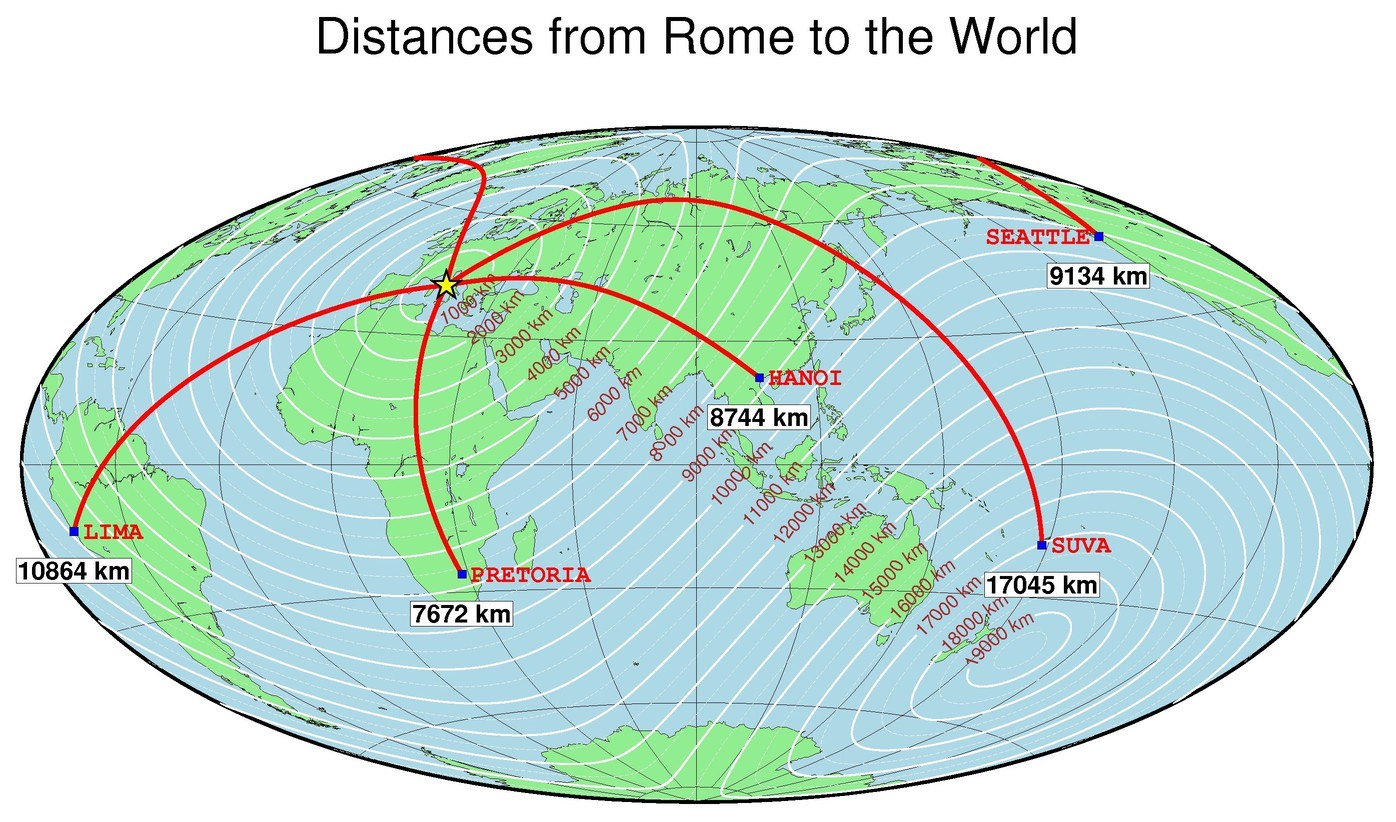## Gmt advanced tutorial, part ii (codes included)

Plotting publishable quality maps using generic mapping tools (GMT)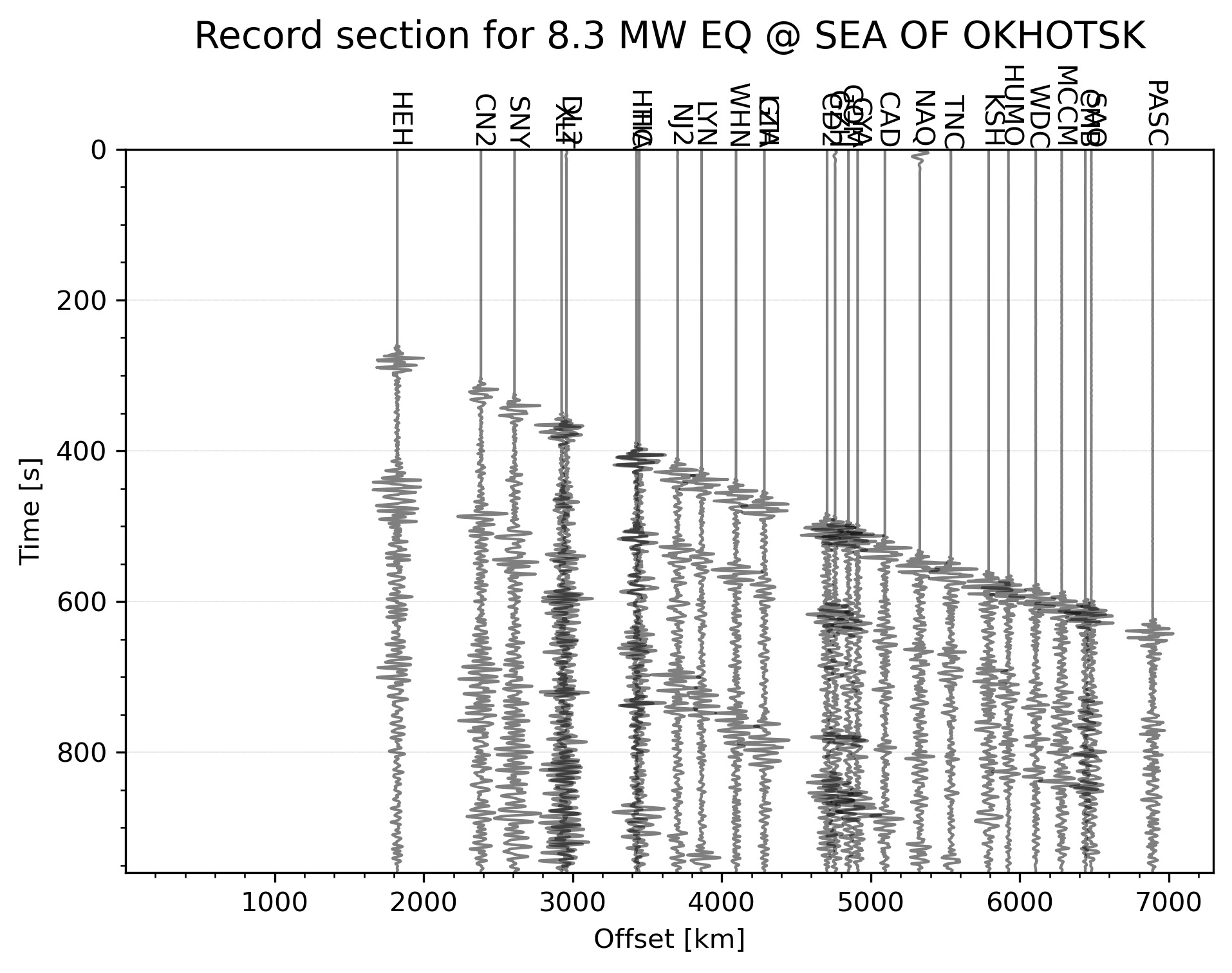## Automatically plotting record section for an earthquake in the given time range in python (codes included)

Python code to automatically plot the record section for the highest magnitude earthquake in the given time range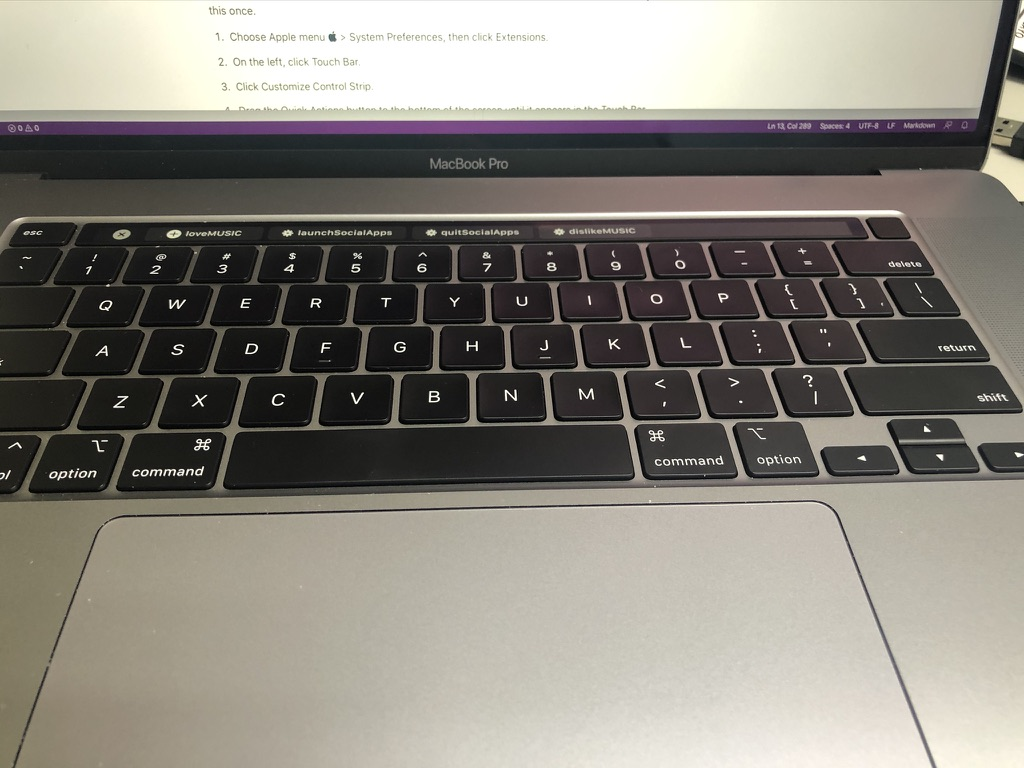## Mac quick action to rate the songs in apple music app [macos]

Quick action for mac to easily love, dislike, rate songs in apple music app## Numerical tests on travel time tomography [matlab]

Introduction to the concepts of tomography with equations and codes. Introduction to the concepts of overdetermined, underdetermined and mix-determined probl...## Monte carlo simulation to test for the correlation between two dataset in matlab (codes included)

We test for the correlation coefficients or the covariance between two sets of random numbers selected from normal distribution using the Monte Carlo simulat...## Easy statistical analysis using the tools of matlab (codes included)

Visualize the statistics of the data using MATLAB: mean, median, std, interquartile range, skewness, kurtosis, t-statistic, degrees of freedom## Hypothesis test in matlab (codes included)

Using Randomization to test the disprove the null hypothesis## Topographic map clipped by coastlines in python (codes included)

This post demonstrate how to use Python to set up clip topographic map based on coastlines.## Topographic map with shading in python (codes included)

Generating GMT style shaded relief map in Python## Plotting the geospatial data clipped by coastlines in python (codes included)

In geosciences, we most frequently have to make geospatial plots, but the available data is unevenly distributed and irregular. We like to show the data, in ...## How to plot topographic station map in python (codes included)

We read the station information from a file to plot the 1 arc-minute relief map## Non-linear curve fitting to a model with multiple observational variables in matlab (codes included)

How to fit data to non-linear model## Plotting 1 arc-minute global relief map in python (codes included)

Plotting 1 arc-minute topographic map in python## Estimation of the degrees of freedom of time series in python (codes included)